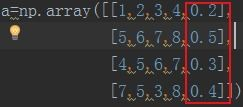# python的argsort

## 1.python argsort（)究竟如何返回的

>>> import numpy

>>> help(numpy.argsort)

Help on function argsort in module numpy.core.fromnumeric:

argsort(a, axis=-1, kind='quicksort', order=None)

Returns the indices that would sort an array.

Perform an indirect sort along the given axis using the algorithm specified

by the `kind` keyword. It returns an array of indices of the same shape as

`a` that index data along the given axis in sorted order.

Examples

--------

>>> x = np.array([3, 1, 2])

>>> np.argsort(x)

array([1, 2, 0])

--------

argsort函数返回的是数组值从小到大的索引值

[3, 1, 2]从小到大为[1,2,3]，期对应的索引为[1,2,0]

## 2.python sort（)用法

Python中的sort（)方法用于数组排序，下面以实例形式对此加以详细说明：

x = [4, 6, 2, 1, 7, 9]x.sort()

print x # [1, 2, 4, 6, 7, 9]如果需要一个排序好的副本，同时保持原有列表不变，怎么实现呢

x =[4, 6, 2, 1, 7, 9]

y = x[ : ]

y.sort()

print y #[1, 2, 4, 6, 7, 9]

print x #[4, 6, 2, 1, 7, 9]注意：y = x[：] 通过分片操作将列表x的元素全部拷贝给y，如果简单的把x赋值给y:y = x,y和x还是指向同一个列表，并没有产生新的副本。

x =[4, 6, 2, 1, 7, 9]

y = sorted(x)

print y #[1, 2, 4, 6, 7, 9]

print x #[4, 6, 2, 1, 7, 9]sorted返回一个有序的副本，并且类型总是列表，如下：

print sorted('Python') #['P', 'h', 'n', 'o', 't', 'y']二、自定义比较函数

def comp(x, y):

if x < y:

return 1

elif x > y:

return -1

else:

return 0

nums = [3, 2, 8 ,0 , 1]

nums.sort(comp)

print nums # 降序排序[8, 3, 2, 1, 0]

nums.sort(cmp) # 调用内建函数cmp ，升序排序

print nums # 降序排序[0, 1, 2, 3, 8]三、可选参数

sort方法还有两个可选参数：key和reverse

1、key在使用时必须提供一个排序过程总调用的函数：

x = ['mmm', 'mm', 'mm', 'm' ]

x.sort(key = len)

print x # ['m', 'mm', 'mm', 'mmm']2、reverse实现降序排序，需要提供一个布尔值：

y = [3, 2, 8 ,0 , 1]

y.sort(reverse = True)

print y #[8, 3, 2, 1, 0]

## 3.Python里的sort语句

Python中的sort（)函数是序列的内部函数，函数原型：

L.sort(cmp=None, key=None, reverse=False)函数作用：它是把L原地排序，也就是使用后并不是返回一个有序的序列副本，而是把当前序列变得有序。

Python中sort（)参数说明：

(1) cmp参数

cmp接受一个函数，拿整形举例，形式为：

def f(a,b):

return a-b如果排序的元素是其他类型的，如果a逻辑小于b，函数返回负数；a逻辑等于b，函数返回0;a逻辑大于b，函数返回正数就行了。

(2) key参数

key也是接受一个函数，不同的是，这个函数只接受一个元素，形式如下：

def f(a):

return len(a)key接受的函数返回值，表示此元素的权值，sort将按照权值大小进行排序

(3) reverse参数

Python中sort（)函数举例：

(1)按照元素长度排序

L = [{1:5,3:4},{1:3,6:3},{1:1,2:4,5:6},{1:9}]

def f(x):

return len(x)

sort(key=f)

print L

//输出：

//[{1: 9}, {1: 5, 3: 4}, {1: 3, 6: 3}, {1: 1, 2: 4, 5: 6}](2)按照每个字典元素里面key为1的元素的值排序

L = [{1:5,3:4},{1:3,6:3},{1:1,2:4,5:6},{1:9}]

def f2(a,b):

return a-b

L.sort(cmp=f2)

print L

//输出：

//[{1: 1, 2: 4, 5: 6}, {1: 3, 6: 3}, {1: 5, 3: 4}, {1: 9}]

## 4.python的lambda形式的理解

lambda 创建一个匿名函数。冒号前面是传入参数，后面是一个处理传入参数的单行表达式。

list的sort 同于内建函数sorted。它有三个参数cmp,key,reverse，均有默认值。

cmp是一个比较器，自定义比较器的话传入两个参数x,y，返回值限制为1(x>y), 0(x=y), -1(x<y)。默认是常规比较，数字比较，字符串比较等等。

key是对序列元素处理（比如问题中序列元素仍是序列，就可以再对序列取索引值），也就是sort的比较器实际接收到值是key处理后的结果（问题中实际参与比较的是1,2,3,4）。

reverse默认是False，意思是从小到大排序，传入True，就从大到小排。

## 5.python中numpy.argmin什么意思

numpy.argmin表示最小值在数组中所在的位置

a = [[1, 4, 2], [3, 4, 5]]

b = np.argmin(a)

a = [[4, 4, 2], [3, 4, 5]]

b = np.argmin(a)

#若有重复只显示第一个最小值的位置

a = [[0, 0, 2], [3, 4, 5]]

b = np.argmin(a)

------------------------------------

axis=0 表示列方向上的最小值索引，axis=1表示行方向的最小值索引

a = [[2, 0, 5], [3, 4, 1]]

b = np.argmin(a, axis=0)

a = [[2, 0, 5], [3, 4, 1]]

b = np.argmin(a, axis=1)

## 6.python的一个sort函数不知道哪里错了

>>> from datetime import datetime

>>> import re

>>> s = '@ 2014 2 14 12:33:52 Boris "load " 0.01'

>>> r = re.compile('^@\s+(\d{4}\s+\d{1,2}\s+\d{1,2}\s+\d+:\d+:\d+)')

>>> datetime.strptime(r.match(s).group(1), '%Y %m %d %H:%M:%S')

datetime.datetime(2014, 2, 14, 12, 33, 52)